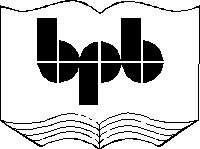3

Variables

This chapter deals with variables. It delves on the various aspects of variables that are available in WMLScript. It starts with the absolute basics of variables i.e. types of variables, creating variables, intialising them, converting a variable of one type to another etc.; and thereafter, the chapter progresses onto the subtleties of using the various variables and explains their related syntactical nuances.

In WMLScript, variables have to be created before they can be used. To create a variable, you simply say var and then you mention the name of the variable. If the variable is not created, then you will not be allowed to use it. At the time of creation of a variable, there is no way of defining what data the variable will hold. That is precisely why WMLScript is said to be a typeless programming language.  The variables have no type.

Lets take the first example. Here we have a variable aa and we make it equal to 100.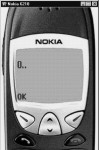Screen 3. 1

aaa.wmls

extern function abc()

{

var aa,bb ;

aa = 100;

aa = typeof(aa);

bb = String.format("%d..",aa);

}

In the above example, we have used typeof(aa). The word typeof is not a function. It is an operator. Therefore, you don't have to use the brackets i.e. ( ). You could have simply used typeof aa. Typeof  returns the data type of the variable.  You may tend to conclude that all variables are typeless !!!. The designers of the WMLScript couldn't make up their mind. So they decided to leave all the variables typeless as far the programmers are concerned. Thus, the programmers simply have to use var to create a variable. The type of the variable depends upon how it has been created or the value that it has been initialized to at the time of creation. Internally there are 5 basic types of variables. The typeof operator determines as to what the data type of the variable is.

The five basic types are as follows :

· Integer (whole numbers)

· Floating point (numbers with decimal places)

· Letters of the alphabet

· Boolean (true or false)

· Invalid - (the value contained in the variable is invalid and it can't be used)

A variable in a WMLScript will always have one of these data types. It can get the data type by one of these means :

· By being initialised to a certain type.

· By being passed as a parameter to a function.

· On being used with an operator like *, /, + , - .

It then gets converted to that data type.

At this point in time, when we execute typeof, it returns 0 because aa has the value 100. When you use String.format, the value of aa is 0. Typeof gives 0 for integers.

aaa.wmls

extern function abc()

{

var aa,bb ;

aa = 100;

aa = typeof(aa);

bb = String.format("%d..",aa);

aa = 100.0;

aa = typeof(aa);

bb = String.format("%d..",aa);

}Screen 3. 2Screen 3. 3

In this example, we first have the value of 100. The typeof will return 0 and then aa gets initialized to 100.0. The typeof will now return a value of 1. This proves that floating points have a type of 1.

When you put anything in double quotes, it is considered a string. The typeof returns a value of 2 for a string.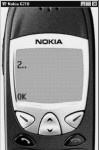Screen 3. 4

aaa.wmls

extern function abc()

{

var aa,bb ;

aa = "AA";

aa = typeof(aa);

bb = String.format("%d..",aa);

}

Boolean means true or false. The words true and false are reserved words in WMLScript. The return value of typeof will now be 3.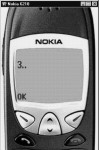Screen 3. 5

aaa.wmls

extern function abc()

{

var aa,bb ;

aa = true;

aa = typeof(aa);

bb = String.format("%d..",aa);

}

A variable can be assigned an invalid value by simply assigning it invalid values without double quotes. An invalid variable can simply never be used.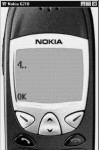Screen 3. 6

aaa.wmls

extern function abc()

{

var aa,bb ;

aa = invalid;

aa = typeof(aa);

bb = String.format("%d..",aa);

}

Now we shall elucidate the method of converting a variable of one data type to another. The rest of this chapter will be used to explain as to how WMLScript achieves this conversion.

In one of the earlier programs, we started by saying aa=100. If you use typeof, it returns a value of 0. Then we changed the value to 100.00. Thus, we changed the data type of aa to a floating point number. At this point, typeof returns a value of 1. This demonstrates that internally, WMLScript not only keeps track of all the variables, but also their current data type.

Where do we use variables ?

(a) The first place is in functions, where we call functions from a library. One function can call another function and you may pass it with parameters.

(b) The next place is with operators like + - * /. Operators would expect a certain data type. The + obviously doesn't need a Boolean or a string. It takes a number and number here means either a number without decimal places, which is an integer, or a number with decimal places, which is a float.

The library String has a function called length. This function accepts a string and it returns back a number equaling the length of the string.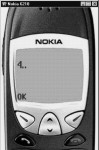Screen 3. 7

aaa.wmls

extern function abc()

{

var aa,bb ;

aa = String.length("ABCD");

bb = String.format("%d..",aa);

}

In this case, the length of the string is 4 because it has 4 characters.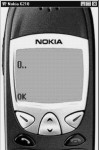Screen 3. 8

aaa.wmls

extern function abc()

{

var aa,bb ;

aa = String.length("");

bb = String.format("%d..",aa);

}

In this example, the answer will be 0.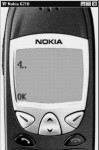Screen 3. 9

If you put a number such as 3290 in double quotes, it will return a value of 4. If you remove the double quotes, String.length will still return the value of 4.

aa = String.length("3290");

aa = String.length(3290);

It is obvious that the number is converted into a string and then the function length is called. By default, any parameter that is supplied is supposed to be a string. So, even if you supply a number, WML will first  convert it into a string and then pass it as a parameter to the function length.

String.length(123.12);   -  6

String.length("123.12")  -  6

If the number has decimal places, it is also converted to a string. This means that while converting from a floating point to a string, the length will count the decimal point also as one character. If you pass the Boolean value of true, length converts it into a string and returns a value of 4 and for false, it returns a value of 5.

String.length(true)          - no display

String.length("true")        -4

String.length(false);         -no display

String.length("false");      -5

The function length converts true and false into strings. Unfortunately, this does not work everywhere because, in the Dialogs.alert, if you use true, the Dialog box doesn't show up. This is because here, true is not converted to a string.

If you supply an invalid variable to the function length, it will give you the length of the invalid string. But the invalid variable cannot be converted to any other type.

String.length("invalid")

If you are dealing with strings, then the numbers with or without decimal places get converted in a straight-forward manner. It is equivalent to putting double inverted commas around the numbers. If it is a Boolean value, the same rule applies. It is as if you have put the double inverted commas. This implies that you can pass numbers or boolean values without using the double quotes. The conversion takes place automatically.  A variable with an invalid value will return an invalid type because you can't convert an invalid to anything else. To reiterate the same fact, if a variable is of any of the three types, and if you need to convert it to a string, you can just pass the variable as a parameter to the string function. It will automatically insert the double inverted commas.

In the case of an integer, we are faced with a problem because we have to search for a function that accepts an integer as a parameter. A function generally accepts a parameter and returns a value. After deliberate effort, we finally found a function called random which accepts an integer as a parameter. This function is Lang.random. A function can accept either an integer or a float as a parameter.

aaa.wmls

extern function abc()

{

var aa,bb ;

aa = Lang.random(10);

bb = String.format("%d..",aa);

}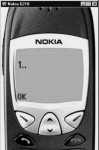Screen 3. 10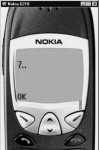Screen 3. 11

Each time you run the program, it will return a random number between 0 and the number you pass to it as a parameter. Run it a few times to confirm this. If you now pass a number with decimal places, such as 10.6, it will convert it from a float to an integer.

aa = Lang.random(10.6);

Even though the documentation says it is not possible, most functions can't convert a float to an int. But there is a library called Float, which has a member called int which throws away the decimal place. So 10.6 becomes 10. Thus, to convert a float into an integer, the function float.int can be used.

The Boolean true has the value of 1 and false has a value of 0. So now, if you use lang.random with true * 10, since the value of true is 1, the value passed to the function will be 1 * 10 = 10.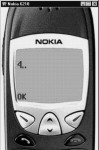Screen 3. 12

aaa.wmls

extern function abc()

{

var aa,bb ;

aa = Lang.random(true*10);

bb = String.format("%d..",aa);

}

On conversion of a Boolean, true is 1, false is 0. The random function converts a string into a number. We will cover this in our next chapter. There is no real difference between an integer and a float as far as this function is concerned. If it is an integer e.g. 10, it will be converted to 10.0. So, effectively there are only 3 data types - boolean, string and numbers.

Now the last conversion is string to boolean. If it is an empty string, it becomes false. For all other values, it is true. Given below are examples with empty strings, if (6) , if (0) , if (-6)  in boolean; numbers which are positive or negative return true , 0 is false and an invalid is always invalid. You cannot convert an invalid to any other type.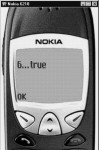Screen 3. 13

aaa.wmls

extern function abc()

{

var aa,bb ;

if (6)

else

}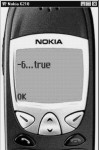Screen 3. 14

aaa.wmls

extern function abc()

{

var aa,bb ;

if (-6)

else

}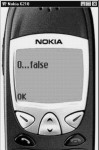Screen 3. 15

aaa.wmls

extern function abc()

{

var aa,bb ;

if (0)

else

}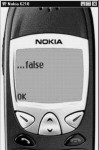Screen 3. 16

aaa.wmls

extern function abc()

{

var aa,bb ;

if ("")